1585035980

# Python For Finance Cryptocurrency Analysis

In this article I will analyze the cryptocurrencies (Litecoin, Ether, & Bitcoin) using the Python programming language. These three currencies are some of the most popular cryptocurrencies in circulation today.

The first thing that I like to do before writing a single line of code is to put in a description in comments of what the code does. This way I can look back on my code and know exactly what it does.

`#Description: This is a python program for cryptocurrency analysis`

Import the libraries.

``````
#Import the libraries
import numpy as np
import pandas as pd

``````

``````

``````

Store the data into dataframes. This data contains a years worth of data from (3/22/2019) to (3/21/2019) for Bitcoin, Ether, and Litecoin.

``````

``````

Print the first 5 rows for all three dataframes.

``````
# Print the data for BTC

``````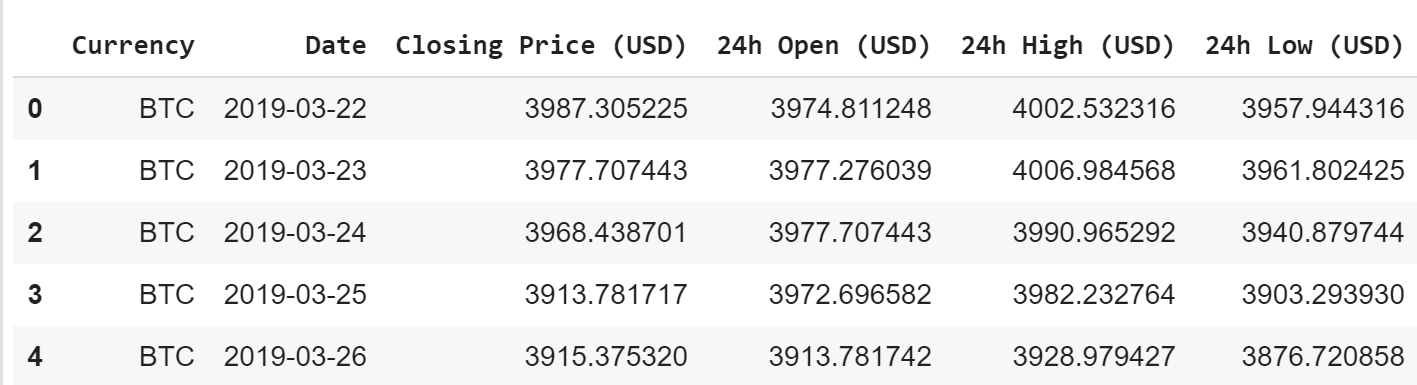``````
# Print the data for ETH

``````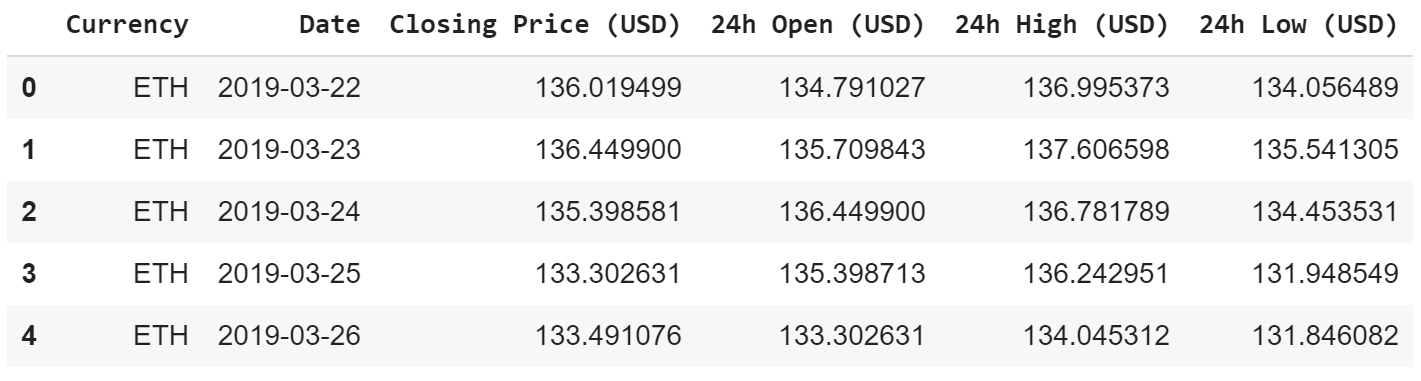``````
# Print the data for LTC

``````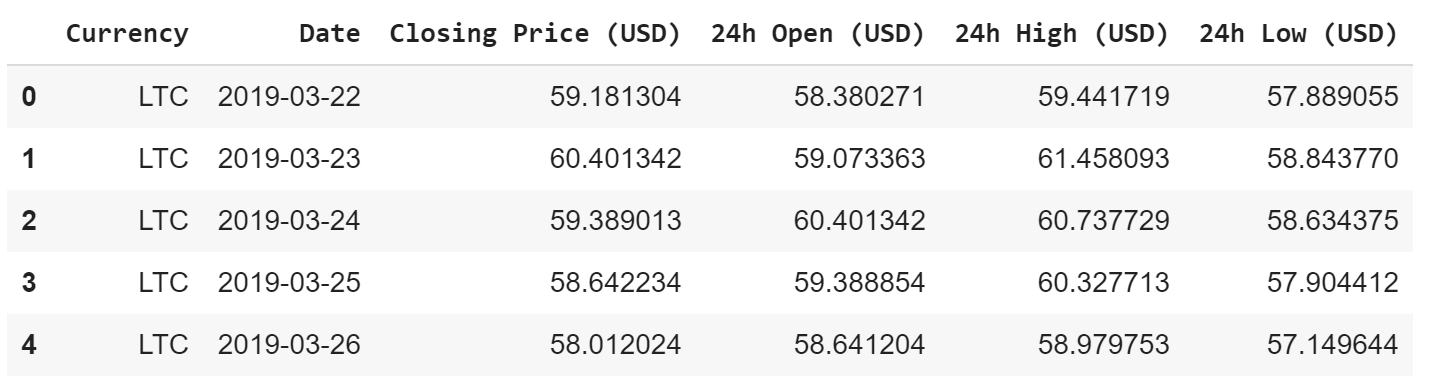Create a new datframe that holds the closing price of all 3 cryptocurrencies.

``````
df = pd.DataFrame({'BTC': df_btc['Closing Price (USD)'],
'ETH': df_eth['Closing Price (USD)'],
'LTC': df_ltc['Closing Price (USD)']
})

``````

Show the new dataframe.

``````
df

``````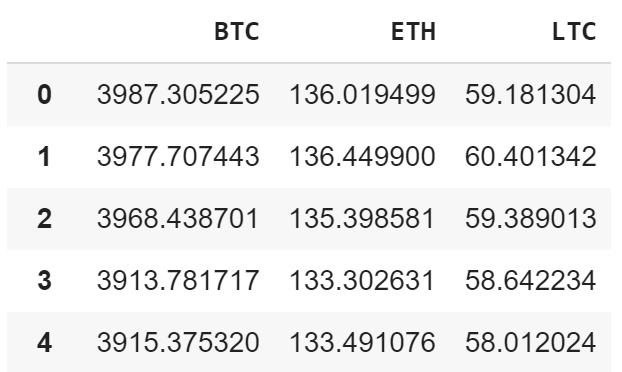Get some statistics on the data.

``````
df.describe()

``````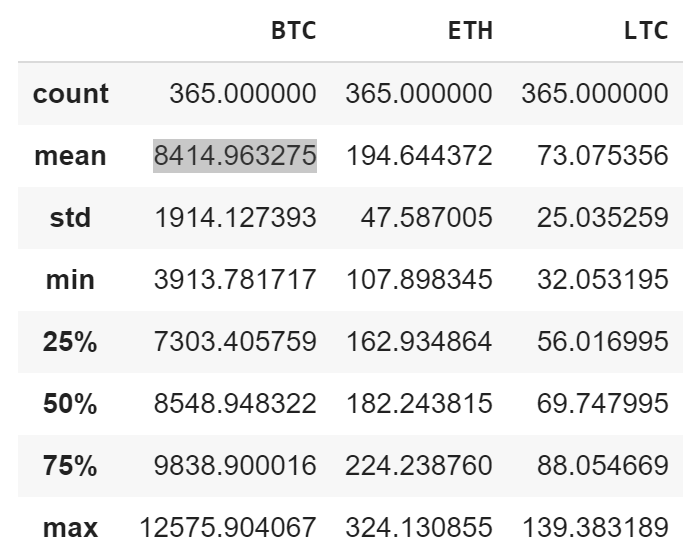Visualize and graph the cryptocurrencies closing price.

``````

import matplotlib.pyplot as plt
plt.style.use('fivethirtyeight')
my_crypto = df
plt.figure(figsize = (12.2, 4.5))
for c in my_crypto.columns.values:
plt.plot(my_crypto[c], label = c)
plt.title('Cryptocurrency Graph')plt.xlabel('Days')
plt.ylabel(' Crypto Price (\$)')
plt.legend(my_crypto.columns.values, loc= 'upper left')
plt.show()

``````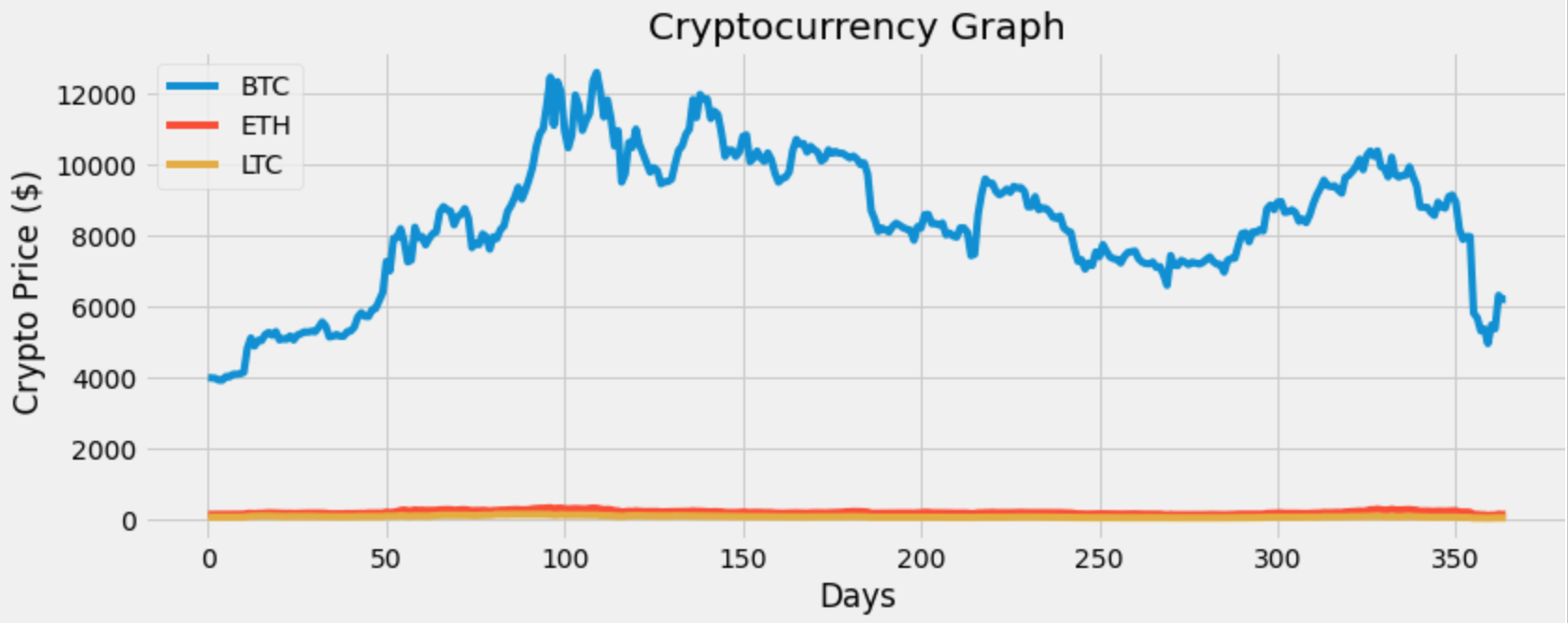The graph above doesn’t truly give us any information on the other two cryptocurrencies (LTC & ETH). So let’s put the data on the same scale and then graph the data.

``````
#Scale the data
#the min-max scaler method scales the dataset so that all the input features lie between 0 and 100 inclusive
from sklearn import preprocessing
min_max_scaler = preprocessing.MinMaxScaler(feature_range=(0, 100))
scaled = min_max_scaler.fit_transform(df)
scaled

``````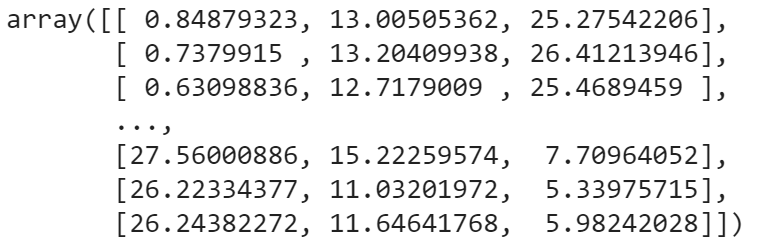Convert the scaled data to a dataframe.

``````
df_scale = pd.DataFrame(scaled, columns = df.columns)

``````

Visualize and graph the scaled data.

``````
#Visualize the scaled data
my_crypto = df_scale
plt.figure(figsize=(12.4, 4.5))
for c in my_crypto.columns.values:
plt.plot(my_crypto[c], label=c)
plt.title('Cryptocurrency Scaled Graph')
plt.xlabel('Days')
plt.ylabel('Crypto Scaled Price (\$)')
plt.legend(my_crypto.columns.values, loc = 'upper left')
plt.show()

``````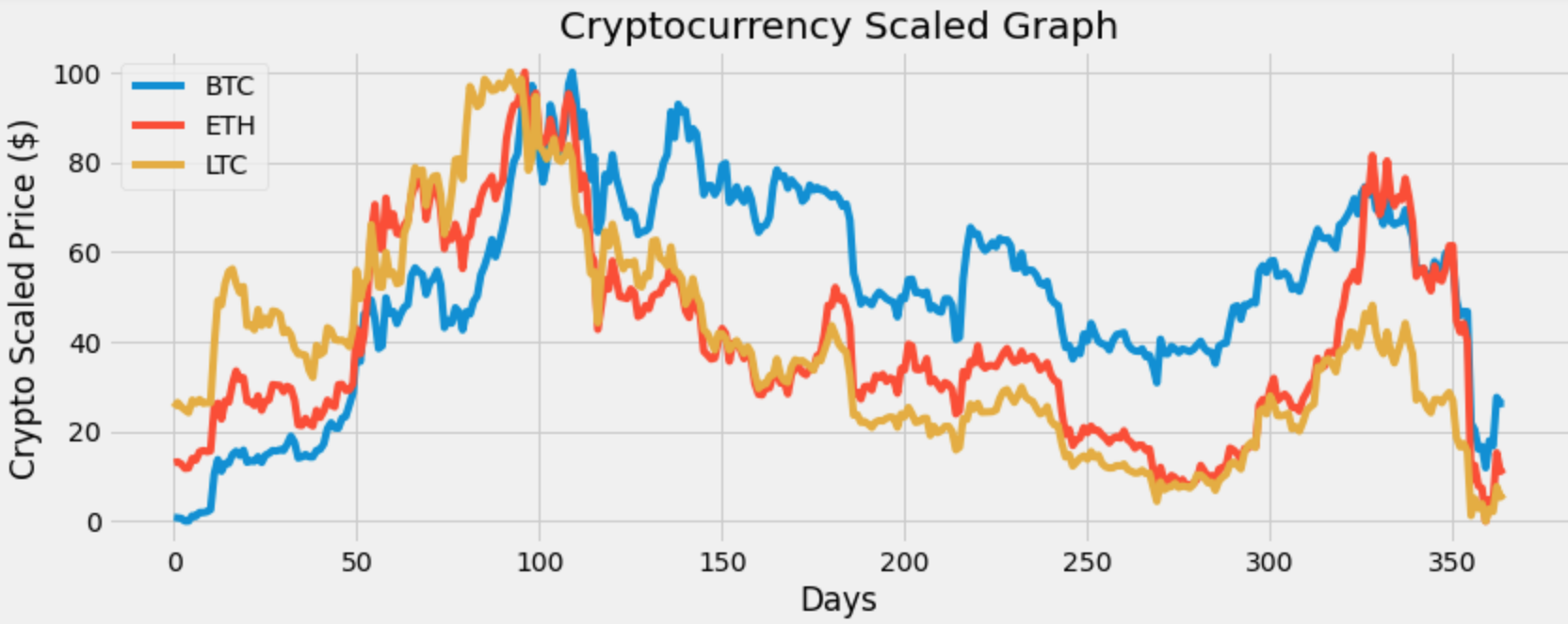That graph looks better. Now I can see that these currencies seem to have a correlation meaning when one asset drops the others drop and when one asset rises the other assets rise. We will check that later.

For now let’s get and show the daily simple return.

``````
DSR = df.pct_change(1)
DSR

``````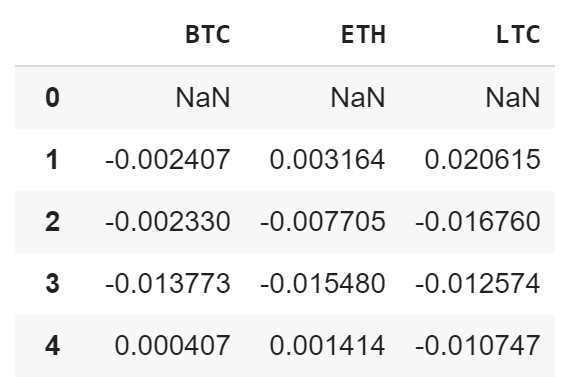Visualize and graph the daily simple returns.

``````
plt.figure(figsize=(12, 4.5))
for c in DSR.columns.values:
plt.plot(DSR.index, DSR[c], label = c, lw = 2, alpha = .7)
plt.title('Daily Simple Returns')
plt.ylabel('Percentage (in decimal form')
plt.xlabel('Days')
plt.legend(DSR.columns.values, loc= 'upper right')
plt.show()

``````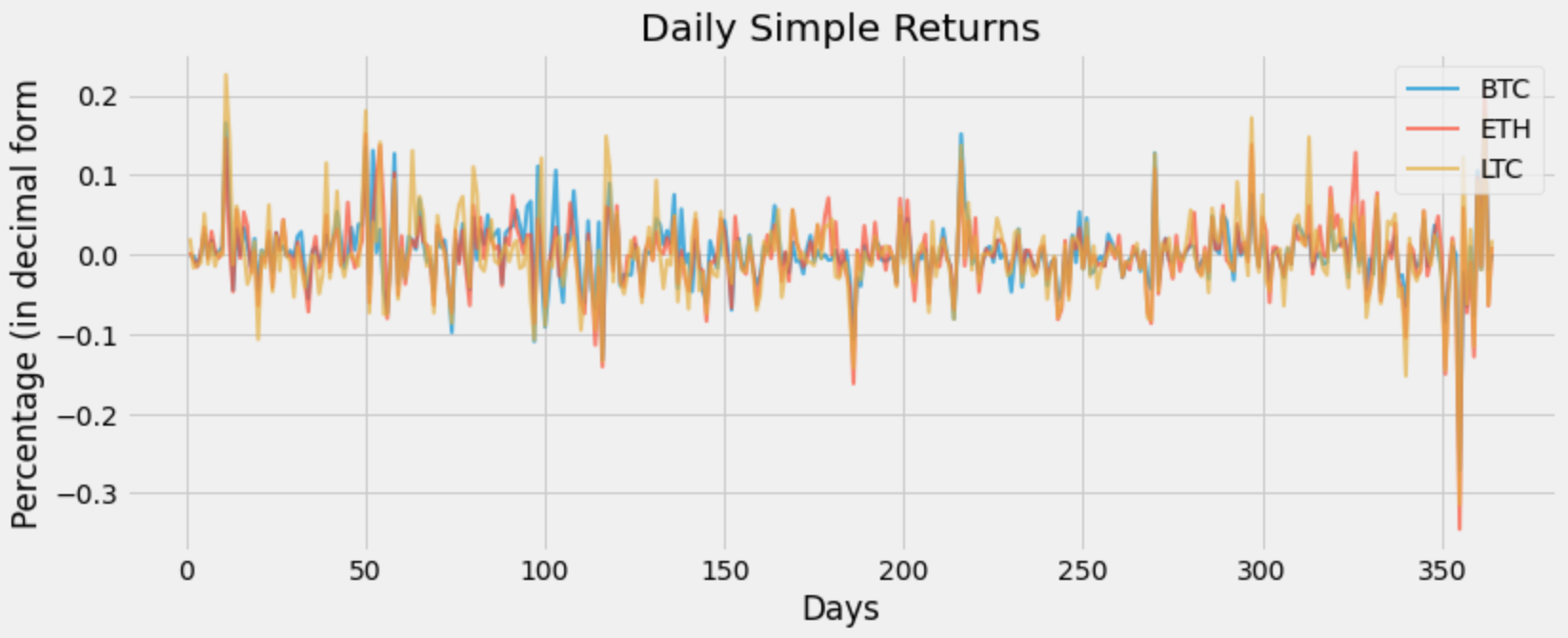Litecoin has some extreme dips in the graph. Let’s take a look at the assets / cryptocurrencies volatility.

``````
print('The cryptocurrency volatility:')
DSR.std()

``````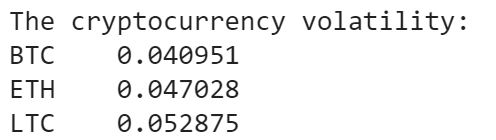The most volatile cryptocurrency is LTC and the least volatile or risky cryptocurrency out of the three is BTC. Show the mean or average daily simple return.

``````DSR.mean()

``````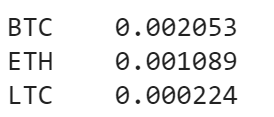So on average I can expect about .2053% return on BTC. Also I noticed that BTC has the highest return and LTC has the lowest return. I did not expect this seeing as how LTC has a higher risk / volatility.

Let’s get the correlation matrix to determine if a change in one variable can result in a change in another.

``````DSR.corr()

``````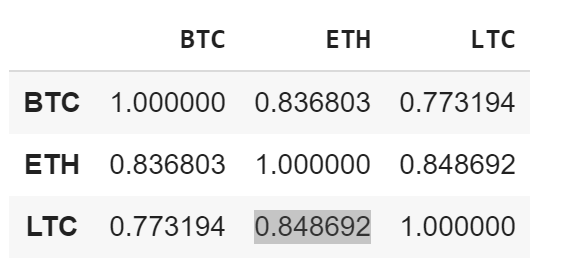Immediately I see that their is a high positive correlation between all three cryptocurrencies. The highest correlation seems to be between ETH and LTC. The currency with the highest correlation to BTC is ETH.

I want to graph this to make it more appealing to the eyes, so I will create a heat map for the correlation matrix.

``````
import seaborn as sns
plt.subplots(figsize= (11,11))
sns.heatmap(DSR.corr(), annot= True, fmt= '.2%')

``````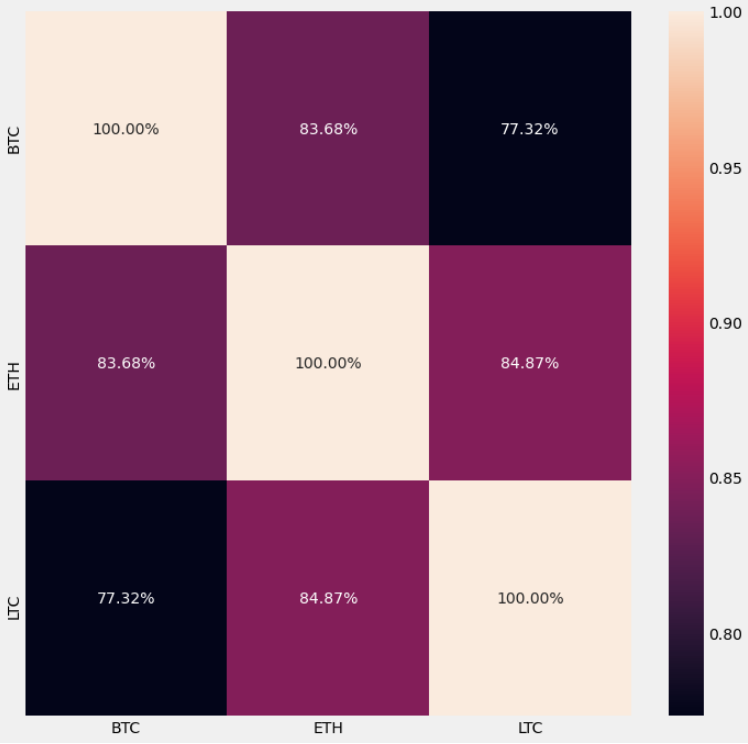Time to get and show the daily cumulative simple returns.

``````
DCSR = (DSR+1).cumprod()
DCSR

``````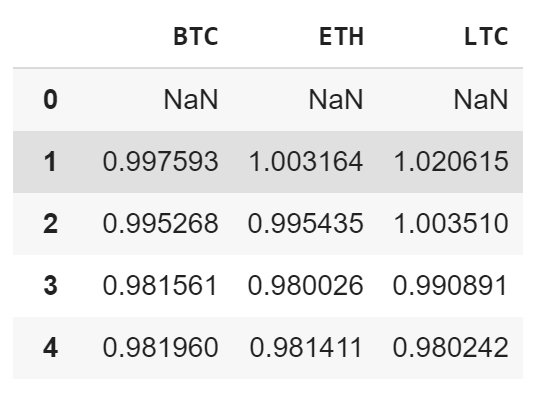Hmm…. Let’s visualize this and see the growth of a \$1 investment in these currencies.

``````
plt.figure(figsize=(12.2, 4.5))
for c in DCSR.columns.values:
plt.plot(DCSR.index, DCSR[c], lw=2, label= c)
plt.title('Daily Cumulative Simple Return')
plt.xlabel('Days')
plt.ylabel('Growth of \$1 investment')
plt.legend(DCSR.columns.values, loc = 'upper left', fontsize = 10)
plt.show()

``````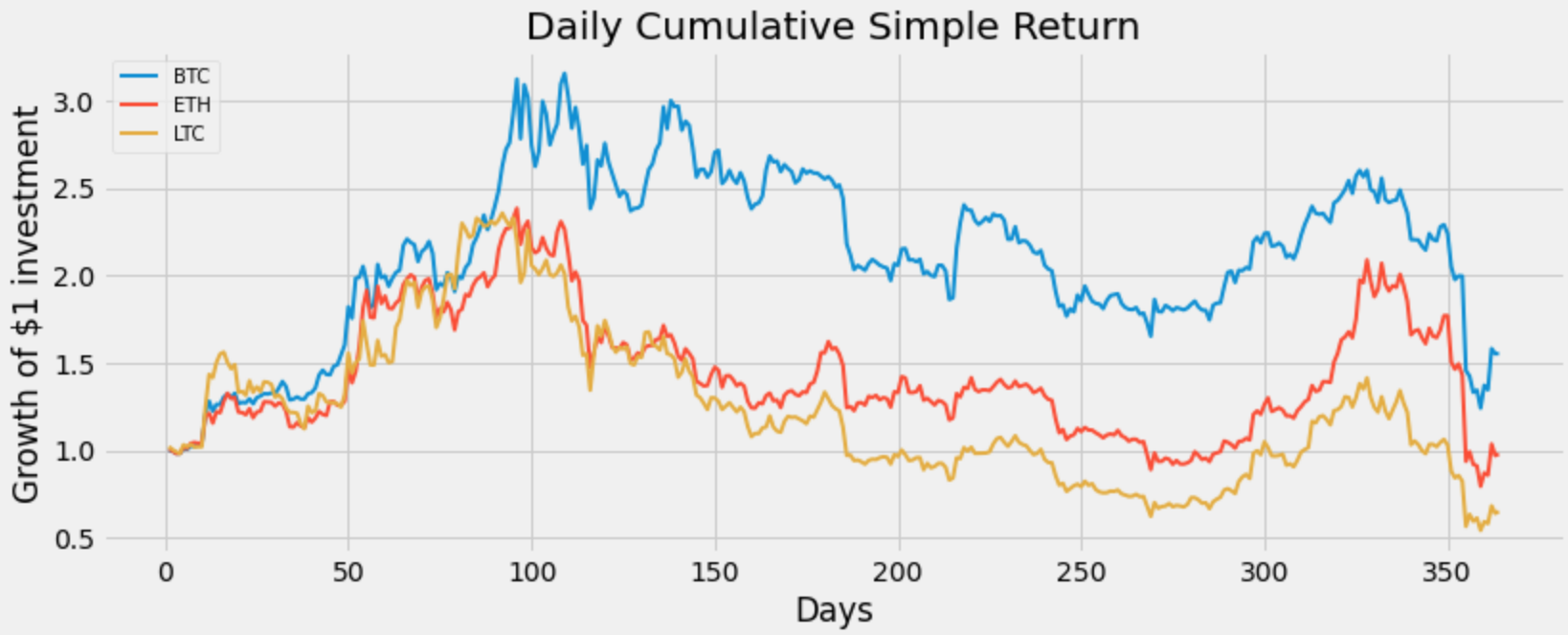Okay looks like if I had invested a dollar in all three assets and sold on day 100, my investment would have grown to about \$2 for LTC, about \$2.20 for ETH, and over \$3.50 for BTC.

Also if I had invested a dollar in all three assets and sold on the last day (day 365), then it looks like I would have lost money in LTC and ETH, however I still would be up in my investment for BTC.

That’s it, we are done creating this program !

#Python #Cryptocurrency

## Buddha Community1619518440

## top 30 Python Tips and Tricks for Beginners

Welcome to my Blog , In this article, you are going to learn the top 10 python tips and tricks.

### 8) Check The Memory Usage Of An Object.

#python #python hacks tricks #python learning tips #python programming tricks #python tips #python tips and tricks #python tips and tricks advanced #python tips and tricks for beginners #python tips tricks and techniques #python tutorial #tips and tricks in python #tips to learn python #top 30 python tips and tricks for beginners1619510796

## Lambda, Map, Filter functions in python

Welcome to my Blog, In this article, we will learn python lambda function, Map function, and filter function.

Lambda function in python: Lambda is a one line anonymous function and lambda takes any number of arguments but can only have one expression and python lambda syntax is

Syntax: x = lambda arguments : expression

Now i will show you some python lambda function examples:

#python #anonymous function python #filter function in python #lambda #lambda python 3 #map python #python filter #python filter lambda #python lambda #python lambda examples #python map1602968400

## Python Tricks Every Developer Should Know

Python is awesome, it’s one of the easiest languages with simple and intuitive syntax but wait, have you ever thought that there might ways to write your python code simpler?

In this tutorial, you’re going to learn a variety of Python tricks that you can use to write your Python code in a more readable and efficient way like a pro.

### Let’s get started

Swapping value in Python

Instead of creating a temporary variable to hold the value of the one while swapping, you can do this instead

``````>>> FirstName = "kalebu"
>>> LastName = "Jordan"
>>> FirstName, LastName = LastName, FirstName
>>> print(FirstName, LastName)
('Jordan', 'kalebu')
``````

#python #python-programming #python3 #python-tutorials #learn-python #python-tips #python-skills #python-development1602666000

## How to Remove all Duplicate Files on your Drive via Python

Today you’re going to learn how to use Python programming in a way that can ultimately save a lot of space on your drive by removing all the duplicates.

### Intro

In many situations you may find yourself having duplicates files on your disk and but when it comes to tracking and checking them manually it can tedious.

Heres a solution

But How do we do it?

If we were to read the whole file and then compare it to the rest of the files recursively through the given directory it will take a very long time, then how do we do it?

The answer is hashing, with hashing can generate a given string of letters and numbers which act as the identity of a given file and if we find any other file with the same identity we gonna delete it.There’s a variety of hashing algorithms out there such as

• md5
• sha1
• sha224, sha256, sha384 and sha512

#python-programming #python-tutorials #learn-python #python-project #python3 #python #python-skills #python-tips1597751700

## How To Compare Tesla and Ford Company By Using Magic Methods in Python

Magic Methods are the special methods which gives us the ability to access built in syntactical features such as ‘<’, ‘>’, ‘==’, ‘+’ etc…

You must have worked with such methods without knowing them to be as magic methods. Magic methods can be identified with their names which start with __ and ends with __ like init, call, str etc. These methods are also called Dunder Methods, because of their name starting and ending with Double Underscore (Dunder).

Now there are a number of such special methods, which you might have come across too, in Python. We will just be taking an example of a few of them to understand how they work and how we can use them.

### 1. init

``````class AnyClass:
def __init__():
print("Init called on its own")
obj = AnyClass()
``````

The first example is _init, _and as the name suggests, it is used for initializing objects. Init method is called on its own, ie. whenever an object is created for the class, the init method is called on its own.

The output of the above code will be given below. Note how we did not call the init method and it got invoked as we created an object for class AnyClass.

``````Init called on its own
``````

Let’s move to some other example, add gives us the ability to access the built in syntax feature of the character +. Let’s see how,

``````class AnyClass:
def __init__(self, var):
self.some_var = var